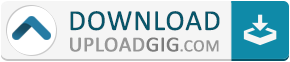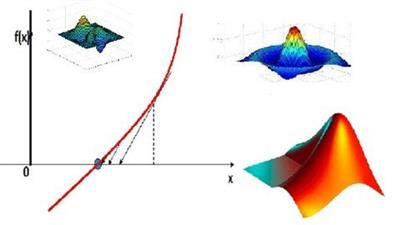#Do not remember me!

### Categories» » » Numerical Methods using MATLAB 2021

## Numerical Methods using MATLAB 2021MP4 | Video: h264, 1280x720 | Audio: AAC, 44.1 KHz
Language: English | Size: 1.36 GB | Duration: 3h 7m

NM with MATLAB
What you'll learn
Basics of MATLAB
MATLAB Array
MATLAB Programming
MATLAB Code
Numerical Methods
Requirements
No programming experience required. You will learn everything here
Description
MATLAB is a popular language for numerical computation. MATLAB is a high-performance language for technical computing. MATLAB is an interactive programming environment for scientific computing. MATLAB is heavily used in many technical fields for data analysis, problem solving, and for experimentation and algorithm development. This course introduces students to MATLAB programming, and demonstrate it’s use for scientific computations.
Numerical method is a mathematical tool designed to solve numerical problems. numerical methods gives us approximate results as there is always some assumptions are made.
The package MATLAB (matrix laboratory) provides an environment in which students can learn to programme and explore the structure of the numerical methods.
There are various problems where we do not have analytical solutions available, or whose exact solution is beyond our current state of knowledge. There are also various problems which required too many (or tedious) calculation to solve it by hand. When such problems arise we can exploit numerical methods to reduce the problem to one involving a finite number of unknowns and use a computer to solve the resulting equations. There are many more such situations where analytical methods are unable to produce desirable results. Even if analytical solutions are available, these are not agreeable to direct numerical interpretation.
In this course we will cover
Introduction of MATLAB
Arrays and Matrices
Basics of MATLAB programming
Numerical methods for engineering problems
Use of MATLAB for Numerical methods
Who this course is for:
People who are willing to learn MATLAB.
Engineering students and Professionals.
Beginner MATLAB developersName:* E-Mail: Security Code: *